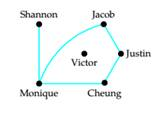# Social Network Each vertex in the graph at the left represents a student. An edge connects two vertices if the corresponding students have at least one class in common during the Current term. Is there a class that both Jacob and Cheung are enrolled in? Who shares the most classes among the members of this group of students? How many classes does Victor have in common with the other students? Is this graph connected?### Mathematical Excursions (MindTap C...

4th Edition
Richard N. Aufmann + 3 others
Publisher: Cengage Learning
ISBN: 9781305965584

#### Solutions

Chapter
Section### Mathematical Excursions (MindTap C...

4th Edition
Richard N. Aufmann + 3 others
Publisher: Cengage Learning
ISBN: 9781305965584
Chapter 5, Problem 1T
Textbook Problem
2 views

## Social Network Each vertex in the graph at the left represents a student. An edge connects two vertices if the corresponding students have at least one class in common during the Current term. Is there a class that both Jacob and Cheung are enrolled in? Who shares the most classes among the members of this group of students? How many classes does Victor have in common with the other students? Is this graph connected?To determine

(a)

To say:

Whether Jacob and Cheung are enrolled in any common classes.

### Explanation of Solution

Given:

Calculation:

Jac...

To determine

(b)

To find:

Which person of this group shares the majority classes

To determine

(c)

To find:

Which person of this group has no communal class with remaining student.

To determine

(d)

To find:

The graph is connected or not.

### Still sussing out bartleby?

Check out a sample textbook solution.

See a sample solution

#### The Solution to Your Study Problems

Bartleby provides explanations to thousands of textbook problems written by our experts, many with advanced degrees!

Get Started

Find more solutions based on key concepts
In problems 23-58, perform the indicated operations and simplify. (x3+x1)(x+2)

Mathematical Applications for the Management, Life, and Social Sciences

In Exercises 47-52, find and simplify f(a+h)f(a)h(h0) for each function. 51. f(x)=1x

Applied Calculus for the Managerial, Life, and Social Sciences: A Brief Approach

In Exercises 7-12, solve for y in terms of x. (y3)2=5+(x+1)2

Calculus: An Applied Approach (MindTap Course List)

Evaluate the integral. 62. d1+cos2

Calculus: Early Transcendentals

Express each ratio in lowest terms: 8048

Elementary Technical Mathematics

In Exercises 1 to 4, find tan and tan for each triangle.

Elementary Geometry for College Students

What characteristic differentiates a pretest-post test design from a time-series design?

Research Methods for the Behavioral Sciences (MindTap Course List)

By logarithmic differentiation, if y=xx+1, then y = a) xx+1(1x1x+1) b) xx+1(1x1x+1) c) xx+1(2x1x+1) d) xx+1(12x...

Study Guide for Stewart's Single Variable Calculus: Early Transcendentals, 8th